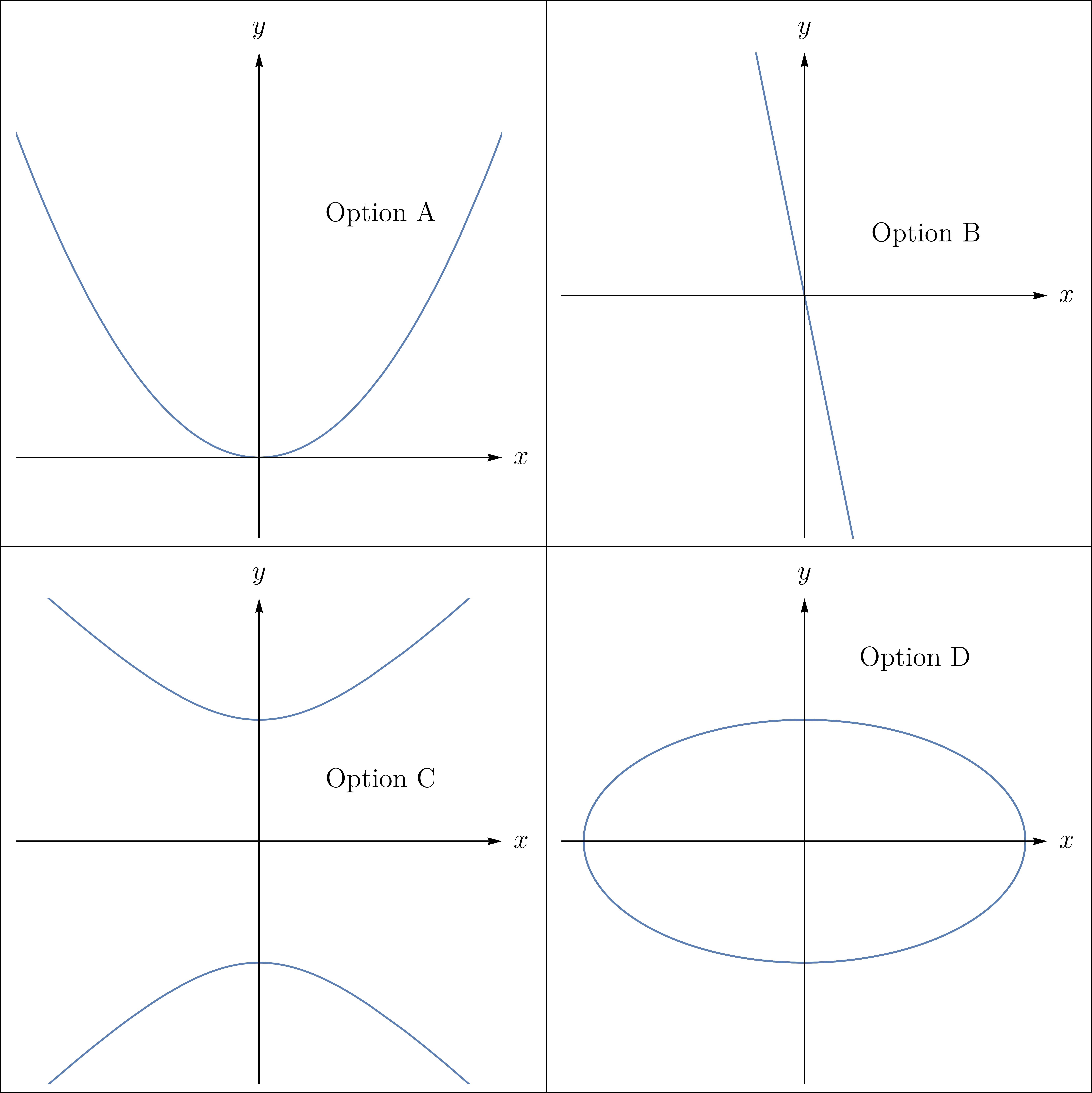Algebra

# Parametric Equations - Graphing

Which of the following is the curve defined by the parametric equations $x=4\sin t, \quad y=8\cos t \ ?$If $(a,b)$ is the center and $r$ is the radius of the circle defined by the parametric equations $x=6\cos 6t, \quad y=6\sin 6t, \quad 0 \leq t \leq 2\pi,$ what is the value of $a+b+r$?

Which of the following is the curve defined by the parametric equations $x=6\sin t, \quad y=5\sin^2 t ?$What shape does the following parametric equation describe:

$x = 3 \cos ^2 \theta, y = 6 \sin^2 \theta.$

Consider the ellipse defined by the parametric equations $x=12\cos t, \quad y=4\sin t,$ where $0 \leq t \leq 2\pi$. If the length of the major axis is $a$ and the length of the minor axis is $b$, what is the value of $a+b$?

×